Info

### # 一、二叉堆概览

``````// 父节点的索引
int parent(int root) {
return root / 2;
}
// 左孩子的索引
int left(int root) {
return root * 2;
}
// 右孩子的索引
int right(int root) {
return root * 2 + 1;
}
``````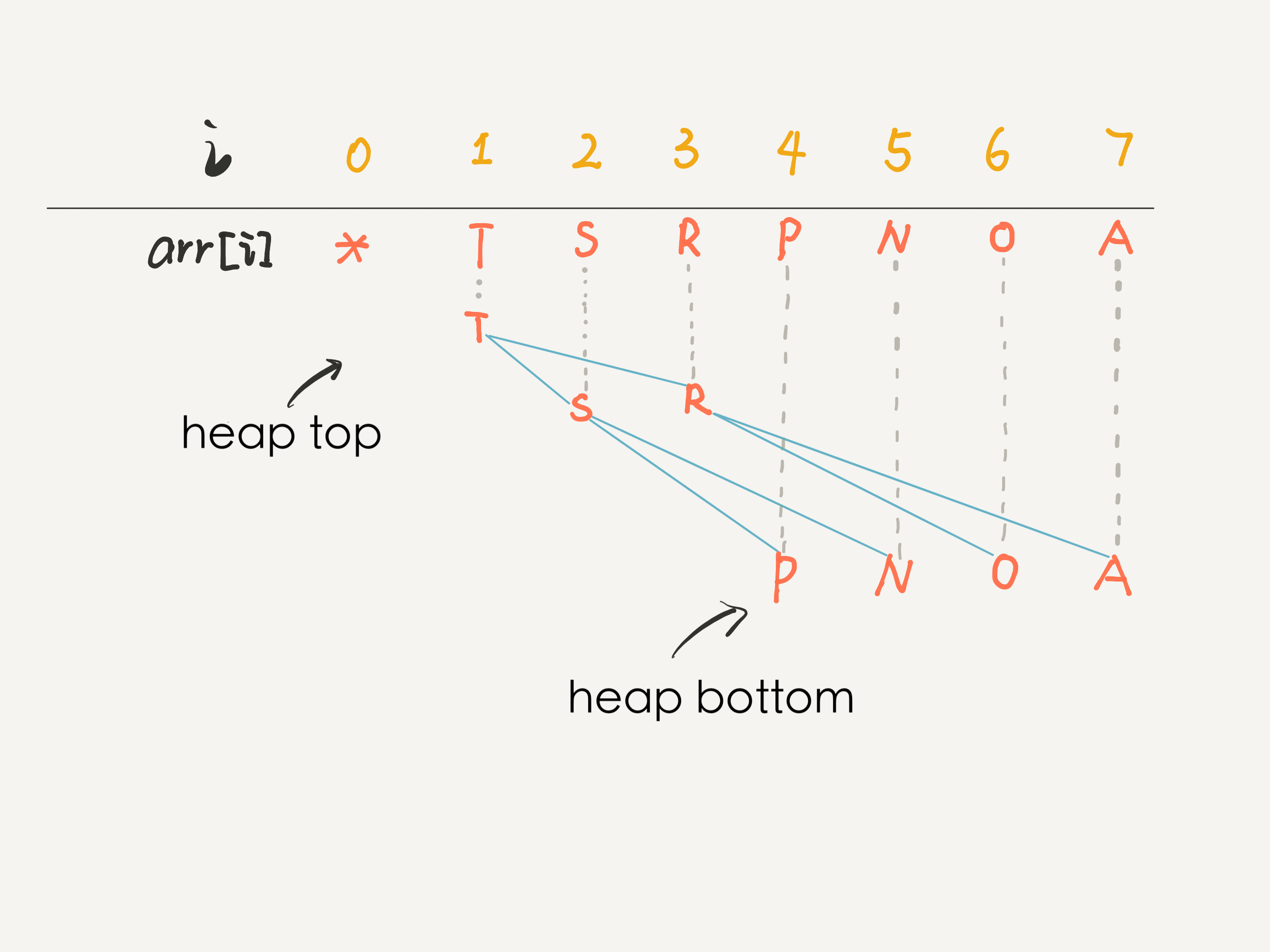### # 二、优先级队列概览

Tip

``````public class MaxPQ
<Key extends Comparable<Key>> {
// 存储元素的数组
private Key[] pq;
// 当前 Priority Queue 中的元素个数
private int size = 0;

public MaxPQ(int cap) {
// 索引 0 不用，所以多分配一个空间
pq = (Key[]) new Comparable[cap + 1];
}

/* 返回当前队列中最大元素 */
public Key max() {
return pq;
}

/* 插入元素 e */
public void insert(Key e) {...}

/* 删除并返回当前队列中最大元素 */
public Key delMax() {...}

/* 上浮第 x 个元素，以维护最大堆性质 */
private void swim(int x) {...}

/* 下沉第 x 个元素，以维护最大堆性质 */
private void sink(int x) {...}

/* 交换数组的两个元素 */
private void swap(int i, int j) {
Key temp = pq[i];
pq[i] = pq[j];
pq[j] = temp;
}

/* pq[i] 是否比 pq[j] 小？ */
private boolean less(int i, int j) {
return pq[i].compareTo(pq[j]) < 0;
}

/* 还有 left, right, parent 三个方法 */
}
``````

### # 三、实现 swim 和 sink

1、如果某个节点 A 比它的子节点（中的一个）小，那么 A 就不配做父节点，应该下去，下面那个更大的节点上来做父节点，这就是对 A 进行下沉

2、如果某个节点 A 比它的父节点大，那么 A 不应该做子节点，应该把父节点换下来，自己去做父节点，这就是对 A 的上浮

``````public class MaxPQ <Key extends Comparable<Key>> {
// 为了节约篇幅，省略上文给出的代码部分...

private void swim(int x) {
// 如果浮到堆顶，就不能再上浮了
while (x > 1 && less(parent(x), x)) {
// 如果第 x 个元素比上层大
// 将 x 换上去
swap(parent(x), x);
x = parent(x);
}
}
}
``````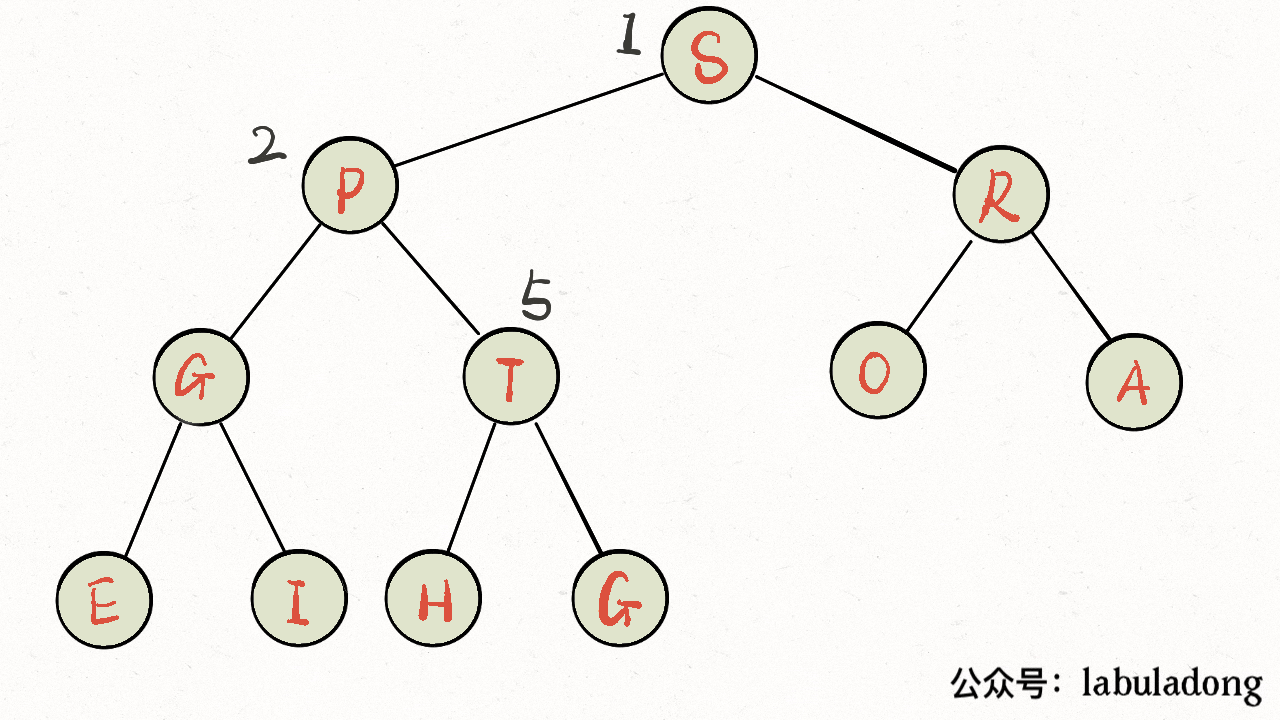``````public class MaxPQ <Key extends Comparable<Key>> {
// 为了节约篇幅，省略上文给出的代码部分...

private void sink(int x) {
// 如果沉到堆底，就沉不下去了
while (left(x) <= size) {
// 先假设左边节点较大
int max = left(x);
// 如果右边节点存在，比一下大小
if (right(x) <= size && less(max, right(x)))
max = right(x);
// 结点 x 比俩孩子都大，就不必下沉了
if (less(max, x)) break;
// 否则，不符合最大堆的结构，下沉 x 结点
swap(x, max);
x = max;
}
}
}
``````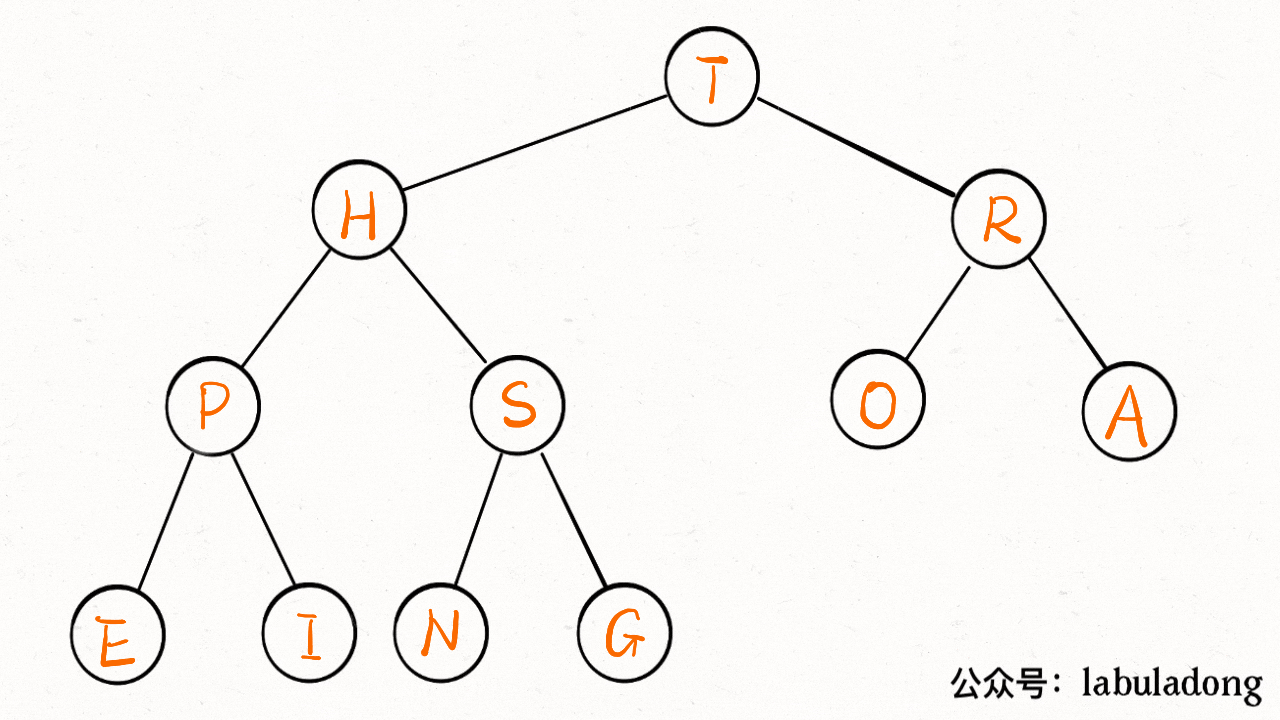### # 四、实现 delMax 和 insert

`insert` 方法先把要插入的元素添加到堆底的最后，然后让其上浮到正确位置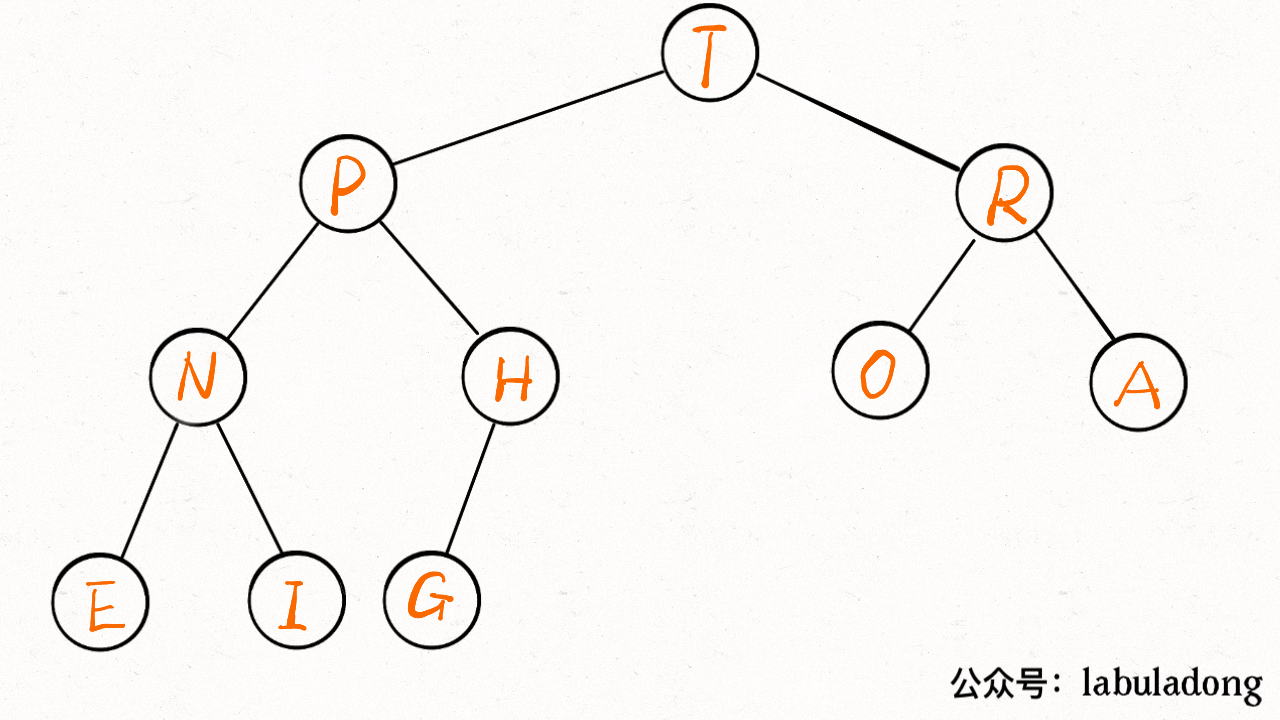``````public class MaxPQ <Key extends Comparable<Key>> {
// 为了节约篇幅，省略上文给出的代码部分...

public void insert(Key e) {
size++;
// 先把新元素加到最后
pq[size] = e;
// 然后让它上浮到正确的位置
swim(size);
}
}
``````

`delMax` 方法先把堆顶元素 `A` 和堆底最后的元素 `B` 对调，然后删除 `A`，最后让 `B` 下沉到正确位置

``````public class MaxPQ <Key extends Comparable<Key>> {
// 为了节约篇幅，省略上文给出的代码部分...

public Key delMax() {
// 最大堆的堆顶就是最大元素
Key max = pq;
// 把这个最大元素换到最后，删除之
swap(1, size);
pq[size] = null;
size--;
// 让 pq 下沉到正确位置
sink(1);
return max;
}
}
``````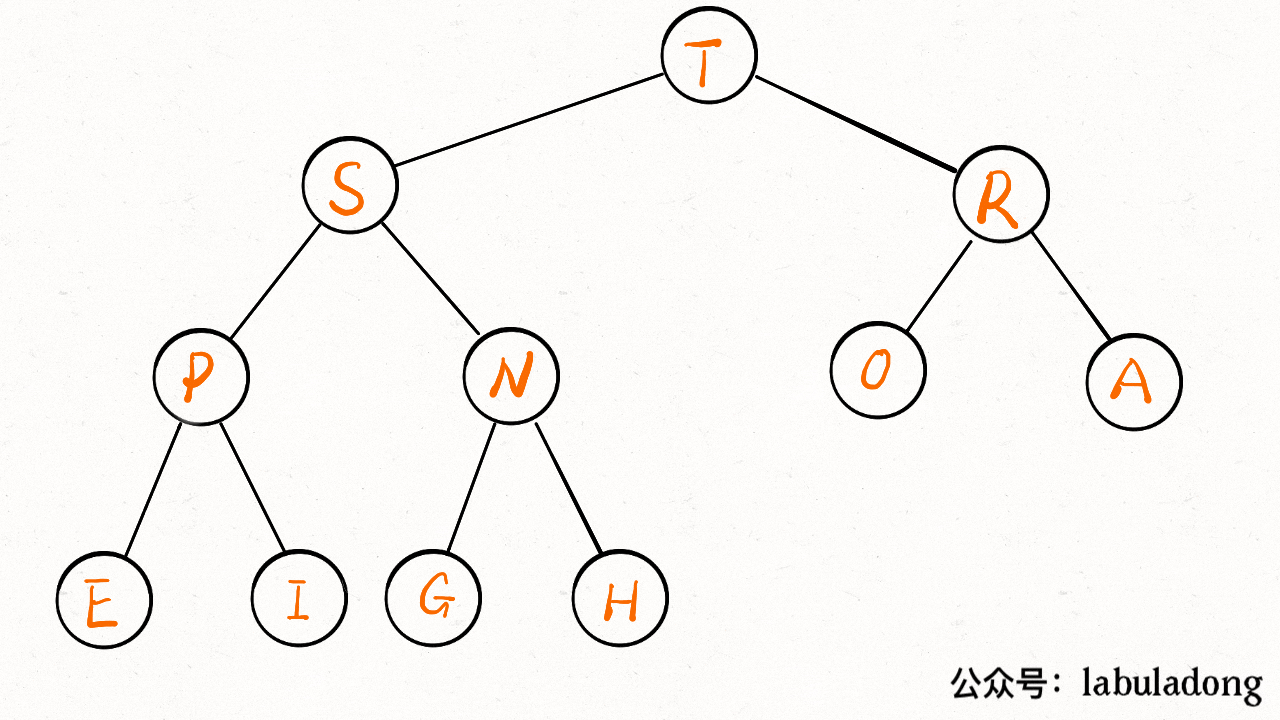### # 五、最后总结